### Home > MC2 > Chapter 9 > Lesson 9.1.1 > Problem9-14

9-14.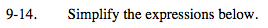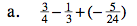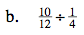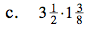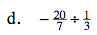Find a common denominator.

$\frac{18}{24}-\frac{8}{24}+\left(-\frac{5}{24}\right)$

Remember, when you divide by a fraction, you are multiplying by its reciprocal.

$\frac{10}{12}\left(\frac{4}{1}\right)=\frac{40}{12}=3\frac{1}{3}$

Before multiplying, convert these mixed numbers into fractions greater than one.

See part (b).

$-8\frac{4}{7}$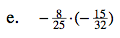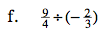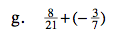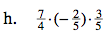Multiply and reduce.

$\frac{-8(-15)}{25(32)}$

$\frac{3}{20}$

See part (b).

See part (a).

$-\frac{1}{21}$

See part (e).

$-\frac{21}{50}$